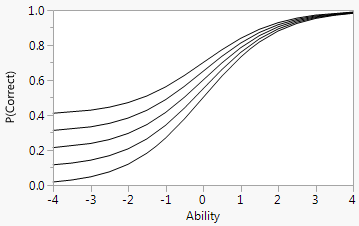Publication date: 08/13/2020

## Item Response Curve Models

One-, two-, and three-parameter logistic models can be used to model the item response curves. The three-parameter logistic (3PL) model is defined as follows.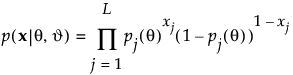P(θ) is the probability of answering the item correctly for an ability level θ. For more information about fitting the item response theory model, see Fitting the IRT Model.

The a parameter defines the steepness of the curve at its inflection point. It provides an estimate of the discriminatory power of the item.

The b parameter defines the location of the inflection point on the Ability axis. It provides an estimate of the difficulty of an item.

The c parameter is the lower asymptote. It provides an estimate of the probability that an item is answered correctly by guessing.

For the 2PL model, the c parameter is set to 0.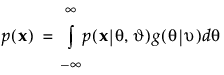For the 1PL model, the c parameter is set to 0 and the a parameter is set to 1. This parameterization is also known as the Rasch model (Rasch 1980).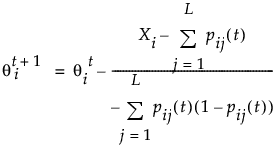### The a Parameter: Item Discrimination

In the 2PL and 3PL models, the a parameter, or the steepness of the curve at its inflection point, provides a measure of the discriminatory power of an item. The discriminatory power, or discrimination, of an item refers to how well an item can distinguish between respondents with low ability levels versus those with high ability levels. A steep item response curve indicates that the item has strong discrimination. Respondents with low ability levels have a low probability of a correct response to the item while respondents with high ability have a high probability of a correct response. Items whose curves are relatively flat have low discrimination. Items with low discrimination are candidates to be dropped from the measurement instrument.

Figure 11.10 Logistic Model for Several Values of a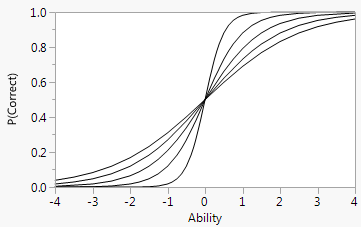### The b Parameter: Item Difficulty

The b parameter, or the location of the inflection point with respect to ability, provides a measure of item difficulty. Item response curves with inflection points farther to the right on the ability scale are indicative of items that are more difficult to answer than items with inflection points to the left. In the 1PL and 2PL models, the b parameter provides an estimate of the ability level required for a 50% probability of correctly answering the item.

Figure 11.11 Logistic Curve for Several Values of b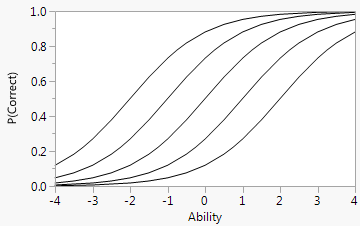### The c Parameter: Guessing

In the 3PL model, the c parameter, or the lower asymptote of the item response curve, provides a measure of the guessing parameter. A nonzero lower asymptote represents the nonzero probability of a person with a very low ability level answering an item correctly.

Figure 11.12 Logistic Model for Several Values of c